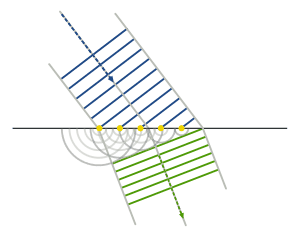# Why does refraction take place ?

Refraction is supposed to take place because there is a difference in the speed of light between the two (or more) media. Why doesn't the light continue in the same direction as before but slower (or faster) ?

Thanks,
Ishan

jtbell
Mentor
Why doesn't the light continue in the same direction as before but slower (or faster) ?

Because then the waves before and after the boundary don't "match" at the boundary.

Refraction is supposed to take place because there is a difference in the speed of light between the two (or more) media. Why doesn't the light continue in the same direction as before but slower (or faster) ?

It does continue in the same direction (at different speed) if it arrives at exactly right angles.

But if the ray of light is slanted one side arrives before the other side and so starts slowing before the second side has arrived.

Just as a vehicle will turn direction if the wheels on one side are slowed by extra resistance eg by rubbing against the kerb, the light ray will bend or be refracted.

At the interface between two media, the fields must obey certain boundary conditions. This follows directly from the Maxwell equations. If the vector perpendicular to the surface boundary is $\hat n$, then, for example,

$$\hat n \cdot D_1 = \hat n \cdot D_2, \quad \hat n \times E_1 = \hat n \times E_2$$

The use of the $D$ field in these conditions that the component of an EM wave that is perpendicular to the surface of the boundary can lengthen and shorten depending on how the permittivity changes. The component that is along the boundary will always stay the same, however. These effects put together constitute refraction.

when you will do electrodynamics ,you will understand that the continuity of the tangential component of E and normal component of D will impose some restriction.

I now understand the Huygens-Fresnel principle but can someone explain how in(at http://en.wikipedia.org/wiki/Huygens–Fresnel_principle)

can we ignore the spherical waves and work with only the wavefronts of the created wave source.